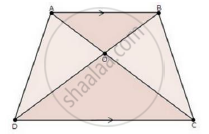Share

# Diagonals Ac and Bd of a Trapezium Abcd with Ab || Dc Intersect Each Other at the Point O. Using Similarity Criterion for Two Triangles, Show that "Oa"/"Oc"="Ob"/"Od" - Mathematics

Course

#### Question

Diagonals AC and BD of a trapezium ABCD with AB || DC intersect each other at the point O. Using similarity criterion for two triangles, show that "OA"/"OC"="OB"/"OD"

#### SolutionWe have,

ABCD is a trapezium with AB || DC

In ΔAOB and ΔCOD

∠AOB = ∠COD                   [Vertically opposite angles]

∠OAB = ∠OCD                   [Alternate interior angles]

Then, ΔAOB ~ ΔCOD         [By AA similarity]

therefore"OA"/"OC"="OB"/"OD"                [Corresponding parts of similar Δ are proportional]

Is there an error in this question or solution?

#### APPEARS IN

RD Sharma Solution for Class 10 Maths (2018 (Latest))
Chapter 7: Triangles
Ex. 7.5 | Q: 9 | Page no. 74
RD Sharma Solution for Class 10 Maths (2018 (Latest))
Chapter 7: Triangles
Ex. 7.5 | Q: 9 | Page no. 74

#### Video TutorialsVIEW ALL 

Solution Diagonals Ac and Bd of a Trapezium Abcd with Ab || Dc Intersect Each Other at the Point O. Using Similarity Criterion for Two Triangles, Show that "Oa"/"Oc"="Ob"/"Od" Concept: Triangles Examples and Solutions.
S